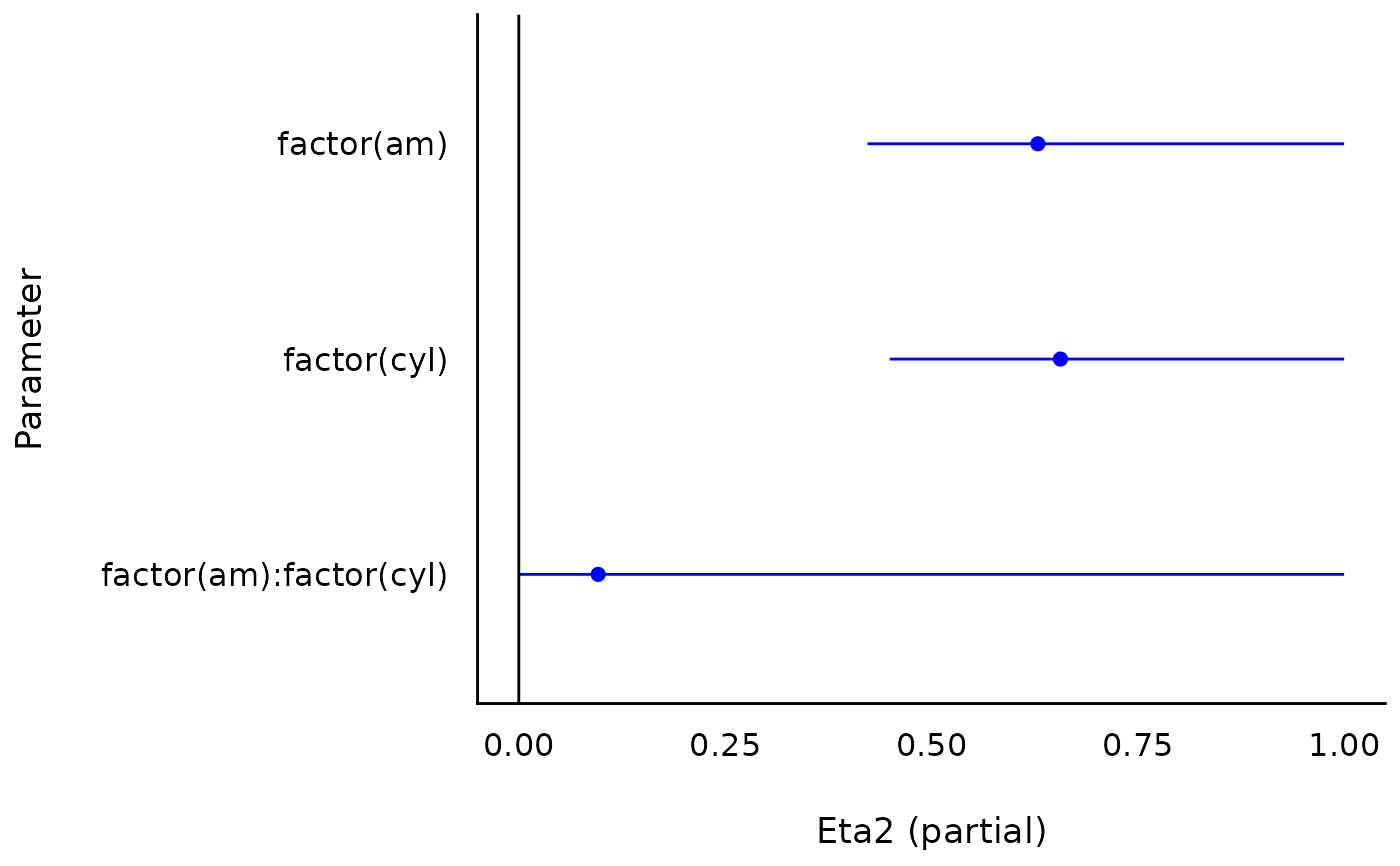The plot() method for the bayestestR::equivalence_test() function.

## Usage

# S3 method for see_equivalence_test_effectsize
plot(x, ...)

# S3 method for see_equivalence_test
plot(
x,
rope_color = "#0171D3",
rope_alpha = 0.2,
show_intercept = FALSE,
n_columns = 1,
...
)

# S3 method for see_equivalence_test_lm
plot(
x,
size_point = 0.7,
rope_color = "#0171D3",
rope_alpha = 0.2,
show_intercept = FALSE,
n_columns = 1,
...
)

## Arguments

x

An object.

...

Arguments passed to or from other methods.

rope_color

Character specifying color of ROPE ribbon.

rope_alpha

Numeric specifying transparency level of ROPE ribbon.

show_intercept

Logical, if TRUE, the intercept-parameter is included in the plot. By default, it is hidden because in many cases the intercept-parameter has a posterior distribution on a very different location, so density curves of posterior distributions for other parameters are hardly visible.

n_columns

For models with multiple components (like fixed and random, count and zero-inflated), defines the number of columns for the panel-layout. If NULL, a single, integrated plot is shown.

size_point

Numeric specifying size of point-geoms.

## Value

A ggplot2-object.

## Examples

library(effectsize)
m <- aov(mpg ~ factor(am) * factor(cyl), data = mtcars)
result <- eta_squared(m)
plot(result)